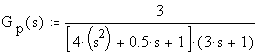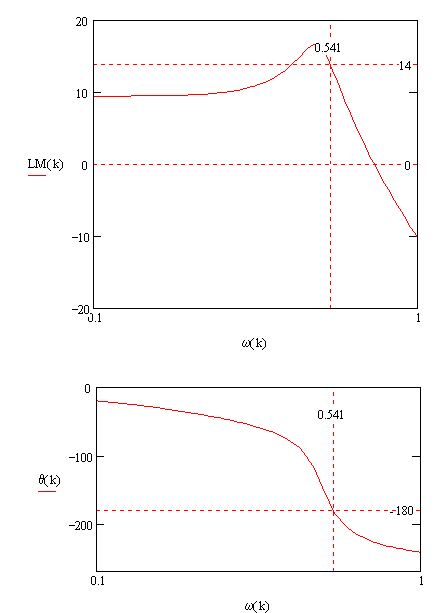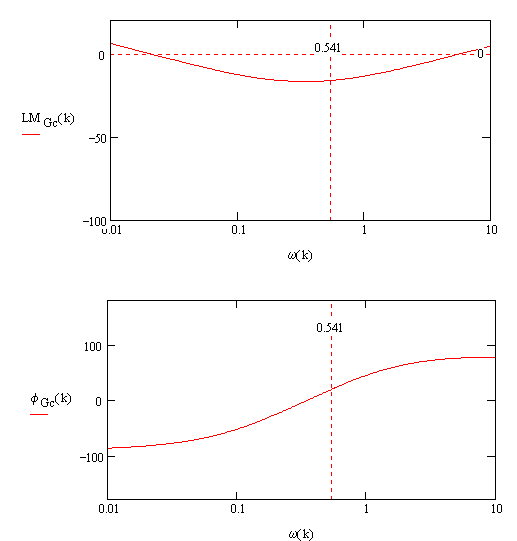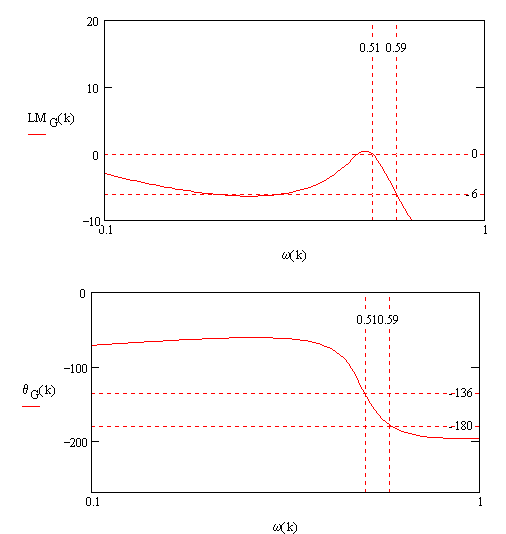Bode Reshaping via PID Control (Ziegler-Nichols Design):
Suppose the process is described by the following transfer function:Using Ziegler-Nichols method, we find that the ultimate gain is given by
Ultimate gain: Ku = 10 -14/20 = 0.2
Ultimate period: Pu = 2p/0.541 = 11.6 sec
Based on the Ziegler-Nichols tuning of parameters, the resulting PID is given bywhere Kc = 0.118, tI = 5.82 and td = 1.454, with a = 0.01. The Bode plots of this PID is given in Figure 2.Figure 2.

Upon connecting Gc in series with Gp, the resulting Bode plots of GcGp are shown in Figure 3.Figure 3.

Note that the result of implementing Ziegler-Nichols yields a gain margin=2 and phase margin approximately 45o.

This page is maintained by Tomas B. Co (tbco@mtu.edu). Last revised 2/13/2000.

Tomas B. Co
Associate Professor
Department of Chemical Engineering
Michigan Technological University
1400 Townsend Avenue
Houghton, MI 49931-1295